# Reverse Synchronous Transmission of Electrical Signals Based on Parallel Injection and Series Pickup

Reverse Synchronous Transmission of Electrical Signals Based on Parallel Injection and Series Pickup
School of Network Engineering, Zhoukou Normal University, Zhoukou 466001, China

School of Computer Science and Technology, Zhoukou Normal University, Zhoukou 466001, China

Corresponding Author Email:
zhanghong@zknu.edu.cn
Page:
655-660
|
DOI:
https://doi.org/10.18280/ts.370415
9 March 2020
|
Accepted:
12 July 2020
|
Published:
10 October 2020
| Citation

OPEN ACCESS

Abstract:

To eliminate the interference with the transmission of electrical signals, this paper puts forward a reverse synchronous transmission (RST) control method based on parallel injection and series pickup. Firstly, the synchronous transmission mechanism of electrical signals was analyzed, followed by the design of the framework and workflow of signal transmission. Next, an RST channel model was established for electrical signals, and the transmission parameters were configured based on the transmission properties of these signals. Through alternative current (AC) impedance analysis, the Laplace transform was performed on the transmission loop to increase the voltage of the transmission channel, and to elevate the signal-to-noise ratio (SNR) of the voltage across the resistor. Finally, the voltage comparator was adopted to obtain the digital information of the baseband signal, and the power signal was transmitted to the RST channel, completing the RST control of electrical signals. The experimental results show that the transmission speed of the system was 0.7488, and the reverse transmission of electrical signals was only delayed by 5ms, when the intensity of electromagnetic radiation was 2.0μT. Through parallel injection and series pickup, the proposed system can effectively realize the RST of electrical signals, without changing the topology of the transmission system.

Keywords:

parallel injection, series pickup, electrical signal, reverse synchronous transmission (RST), alternative current (AC) impedance

1. Introduction

Signal transmission plays an important role in the electricity transmission in inductively coupled power transfer (ICPT) system. The signals can feedback the working conditions or transmit control commands. Traditionally, the ICPT system synchronously transmits electrical signals by three methods: single channel technology, dual channel technology and radio frequency technology. Judging by system integration and reliability, the single channel technology boasts relatively high research value and good application prospects.

Based on signal transmission mode, single channel technology falls into two categories: energy modulation and carrier modulation. In energy modulation, the electrical signals are transmitted by identifying and creating an energy envelope. This transmission mode can be further divided into voltage regulation, frequency modulation and tuning. However, energy modulation suffers from poor electrical quality and low transmission efficiency.

In carrier modulation, the signal spectrum can be expanded through sine wave carrier modulation, facilitating the signal transmission in the channel. Then, the modulated signal is transmitted to the energy channel, which serves as a signal channel for transmission. This modulation method has been widely used and researched, for its limited impact on power transmission. However, the electrical signals need to be transmitted through an independent channel, rather than general channels. Otherwise, the power frequency will interfere with the channel operation, causing errors to signal transmission.

To solve the above problems, this paper proposes a reverse synchronous transmission (RST) method for electrical signals based on parallel injection and series pickup. Firstly, an RST channel was constructed after analyzing the RST process of electrical signals. The transmission parameters of the modulated signal were adjusted through parallel injection and series pick-up. Through alternative current (AC) impedance analysis, the Laplace transform was performed on the transmission loop to increase the voltage of the transmission channel, and to elevate the signal-to-noise ratio (SNR) of the voltage across the resistor. Finally, the signal was demodulated by the voltage comparator to obtain the digital information of the baseband signal, and the power signal was transmitted to the RST channel.

2. RST Mechanism Based on Parallel Injection and Series Pickup

This paper selects a classic ICPT system  as the experimental system. The basic architecture of the system is given in Figure 1.

The ICPT system is divided into a primary side and a secondary side. The current from the DC power supply on the primary side is inverted by high-frequency, transmitted to the receiving coil of the secondary side, and supplied to the electrical equipment after compensation, rectification, and filtering. Take the compensation architecture of series pickup on the primary side and parallel injection on the secondary side for example. The decoupled equivalent circuit  of the architecture is shown in Figure 2. Based on the principle of reflected impedance, the signals are transmitted from the secondary side to the primary side.

The voltage modulation of the primary and secondary loops can be respectively described as:

\left\{ \begin{align} & {{Z}_{11}}{{I}_{1}}-j\omega M{{I}_{2}}={{V}_{1}} \\ & -j\omega M{{I}_{i}}+{{Z}_{22}}{{I}_{2}}=0 \\ \end{align} \right.           (1)

where, Z11 and Z22 are the self-impedances of the primary and secondary loops, respectively. The following can be derived from formula (1):

${{I}_{1}}=\frac{{{V}_{1}}}{j\left( \omega {{L}_{1}}+\omega {{L}_{2}}-\frac{1}{\omega {{C}_{1}}}-\frac{\omega {{C}_{2}}R_{L}^{2}}{1+{{\omega }^{2}}C_{2}^{2}R_{L}^{2}} \right)+\frac{{{R}_{L}}}{1+{{\omega }^{2}}C_{2}^{2}R_{L}^{2}}}$                       (2)

The above analysis shows that the current is affected by the primary-side compensation capacitor , secondary-side compensation capacitor C2, and the load RL. To complete the RST of electrical signals at load change, formulas (1) and (2) were combined to design a control algorithm for the RST of electrical signals in the ICPT system  (Figure 3), which adds a signal conditioning structure and a signal modulation structure to the primary and secondary sides, respectively.

## 1.png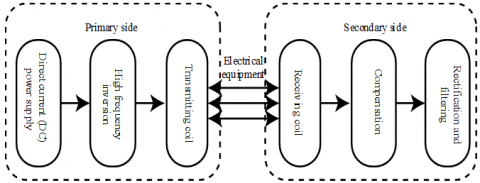Figure 1. The basic architecture of the ICPT system

## 2.png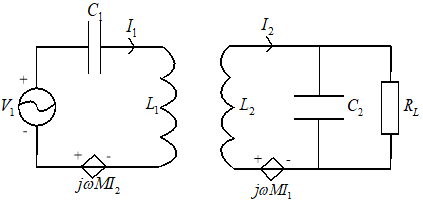Figure 2. The equivalent circuit of the primary and secondary sides of the ICPT system

The RST loop consists of the DC input voltage Ud, a high-frequency inverter composed of S1-S4, the primary-side series pickup capacitor C1, the secondary-side parallel injection capacitor C2, a two-way switch that cuts in/out the signal modulation capacitor C0, a variable load RL, a current energy detection circuit, a drive module, and a signal demodulation structure.

As shown in Figure 3, the DC current is converted into AC current by the high-frequency inverter, and transmitted to the secondary side through inductive coupling, before powering the load via pickup and filtering. The load size is evaluated by the current energy detection circuit. Based on the detected information, the controller [5-9] cuts in/out the signal modulation capacitor C0 via the drive module and the two-way switch, providing the primary-side current with signal features. Finally, the signals are restored and extracted through the signal conditioning structure.

## 3.png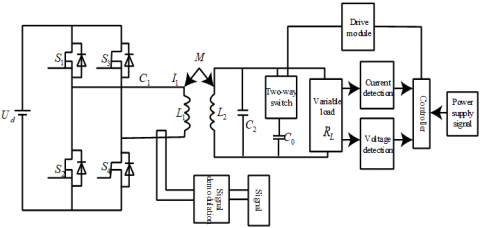Figure 3. The workflow of the RST of electrical signals

3. Modeling of RST Channel

If the architecture and parameters (except for load) of the ICPT are determined, the primary-side currents when the signal modulation capacitor C0 is cut into/out of the system were calculated by the principle of reflected impedance:

(1) When the signal modulation capacitor C0 is cut out of the system, the equivalent circuit diagram of the system is shown in Figure 2. If $\omega^{2} L_{1} C_{1}=1$ and $\omega^{2} L_{2} C_{2}=1$, then the primary-side current can be calculated by:

${{I}_{p1}}=\frac{{{V}_{1}}{{L}_{2}}}{{{\omega }^{2}}{{M}^{2}}C_{2}^{2}{{R}_{L}}}$         (3)

(2) When the signal modulation capacitor C0 is cut into the system, the equivalent circuit diagram of the system is shown in Figure 4.

## 4.png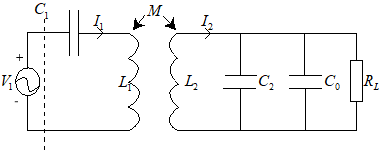Figure 4. The equivalent circuit diagram of the system with C0 cut-in

As shown in Figure 4, the overall impedance of the system can be expressed as:

${{Z}_{2}}={{Z}_{11}}+\frac{{{\left( \omega M \right)}^{2}}}{j\omega {{L}_{2}}+j\omega \left( {{C}_{2}}+{{C}_{0}} \right)}$         (4)

If $\omega^{2} L_{1} C_{1}=1$ and $\omega^{2} L_{2} C_{2}=1$, then the primary-side current can be calculated by:

${{I}_{p2}}=\frac{{{V}_{1}}}{\left| \frac{\omega {{M}^{2}}+{{\omega }^{2}}{{M}^{2}}\left( {{C}_{0}}+{{C}_{2}} \right){{R}_{L}}i}{{{L}_{2}}\left( \omega {{C}_{0}}{{R}_{L}}-i \right)} \right|}$         (5)

It can be seen from the formula (5) that the cut-in and cut-out of signal modulation capacitor C0 has a certain interference to the size of the primary-side current. The selection of C0 interferes with not only the efficiency and transmission power of the system, but also the accuracy of signal demodulation. It is important to rationalize the size of C0 to make the secondary side work near the resonance frequency [10-12]. Taking $C_{0}=0.22 \mu F$ for example, this paper configures the following ICPT system parameters to analyze the control algorithm of the system and the signal modulation strategy under load changes:

The input DC voltage $U_{d} / V$ is 10; the primary-side resonance capacitance $C_{1} / \mu F$ is 0.47; the primary-side resonance inductance $L_{1} / \mu H$ is 134.7; the secondary-side resonance capacitance $C_{2} / \mu F$ is 1.0; the secondary-side resonance inductance $L_{2} / \mu H$ is 63.3; the signal modulation capacitance $C_{0} / \mu F$ is 0.22; The mutual inductance $M / \mu H$ is 18.5.

When the signal modulation capacitor is cut out of or into the circuit, the effective value of the primary-side current will change with the load resistance [13-15] (Figure 5).

## 5.png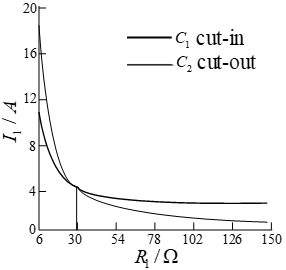Figure 5. The variation of primary-side current with load changes

As shown in Figure 5, the primary-side current curves of C0 cut-in and C0 cut-out intersected at a point, where the resistance is denoted as R0 . If the system architecture and parameters are fixed, R0 is a constant value.

4. Realization of RST of Electrical Signals

4.1 Parameter configuration

The topology of the transmission channel must be clarified before identifying the transmission features of electrical signals . To analyze the transmission channel, a choke coil Lz and a choke capacitor Cz were added to the secondary-side circuit, forming a choke network that satisfies:

${{C}_{z}}=\frac{1}{\omega _{s}^{2}{{L}_{z}}}$       (6)

where, $\omega_{s}$ is the angular velocity of signal transmission.

Under the above parameter configuration, the choke network has a large impedance for high-frequency electrical signals, which is equivalent to an open circuit. In this way, the carrier current to be incorporated into the secondary-side resonant coil to the maximum extent.

To increase the intensity of signal transmission at the transmitting end and reduce the signal attenuation in the channel, this paper takes the secondary-side resonant coil into the analysis of signal resonance. Hence, the transmission capacitance CT of the secondary-side electrical signals can be calculated by:

${{C}_{T}}=\frac{1}{\omega _{S}^{2}\left( {{L}_{S}}+{{L}_{T2}} \right)}$         (7)

To eliminate electrical interference  and increase the received signal strength, a resonant capacitor CR2 was injected in parallel across the secondary-side signal coupling transformer LR2:

${{C}_{R2}}=\frac{1}{\omega _{S}^{2}{{L}_{R2}}}$        (8)

4.2 Transmission process

The transmission process of electrical signals is illustrated in Figure 6, where it is the transmitted current, is is the secondary-side current, iP is the primary-side current, and ir is the parallel pickup current.

## 6.png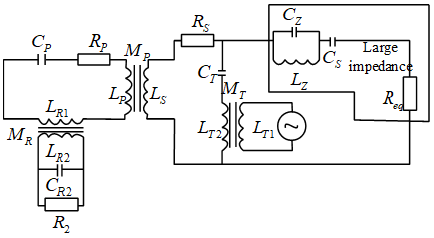Figure 6. The transmission process of electrical signals

As show in Figure 6, the power signals are transmitted in four loops. Loop 1 modulates the electrical signals, and introduces the modulated voltage into the signal coupling transformer, integrating the signals to the main circuit. Loop 2 makes the electrical signals to approach the fully resonant state, and enhances the strength of the signal to a certain extent. Loop 3 is comparable to a frequency selective filter composed of primary-side coil and capacitor, which attenuates the electrical signals of megahertz frequency. Loop 4 make the circuit resonance frequency equal to the frequency of the electrical signals, amplifying the transmission frequency and received signal strength.

4.3 Voltage gain of transmission channel

Through AC impedance analysis, the following can be derived from Figure 6:

\left\{ \begin{align} & {{Z}_{4}}=s{{L}_{R2}}+\frac{{{R}_{2}}}{1+s{{C}_{R2}}{{R}_{2}}} \\ & {{Z}_{43}}=-\frac{{{s}^{2}}M_{R}^{2}}{{{Z}_{4}}} \\ & {{Z}_{3}}=s{{L}_{P}}+{{R}_{p}}+\frac{1}{s{{C}_{P}}}+s{{L}_{R1}}+{{Z}_{43}} \\ & {{Z}_{32}}=-\frac{{{s}^{2}}M_{P}^{2}}{{{Z}_{3}}} \\ & {{Z}_{2}}=sLs+\frac{1}{s{{C}_{T}}}+{{R}_{S}}+s{{L}_{t2}}+{{Z}_{32}} \\ & {{Z}_{21}}=-\frac{{{s}^{2}}M_{T}^{2}}{{{Z}_{2}}} \\ & {{Z}_{1}}=s{{L}_{T1}}+{{Z}_{21}} \\ \end{align} \right.                (9)

where, Zx is the Laplace transform of the equivalent impedance in loop x; Zxy is the Laplace transform of the impedance of the mapping from loop x to loop y, x, y=1, 2, 3, 4, $x \neq y$.

According to Kirchhoff's laws for voltage and current, we have:

\left\{ \begin{align} & {{G}_{1}}\left( \omega \right)=\frac{is}{{{U}_{s}}}=\frac{1}{{{L}_{T1}}\frac{{{Z}_{2}}}{{{M}_{T}}}-s{{M}_{T}}} \\ & {{G}_{2}}\left( \omega \right)=\frac{{{i}_{P}}}{{{i}_{S}}}=\frac{s{{M}_{P}}}{{{Z}_{3}}} \\ & {{G}_{3}}\left( \omega \right)=\frac{{{i}_{r}}}{{{i}_{P}}}=\frac{s{{M}_{R}}}{{{Z}_{4}}} \\ & {{G}_{4}}\left( \omega \right)=\frac{{{U}_{R2}}}{{{i}_{r}}}=\frac{{{R}_{2}}}{1+s{{C}_{R2}}{{R}_{2}}} \\ \end{align} \right.              (10)

Then, the voltage gain of the transmission channel can be obtained as:

$G\left( \omega \right)=\frac{{{U}_{R2}}}{{{U}_{S}}}={{G}_{1}}\left( s \right){{G}_{2}}\left( s \right){{G}_{3}}\left( s \right){{G}_{4}}\left( s \right)$          (11)

4.4 Signal conditioning

Figure 7 presents the architectures of the signal transmitting circuit. In this paper, a Hartley oscillator is used to generate high-frequency sine wave, and a digital multiplexer is employed for signal modulation. To meet the power requirement of the transmitting end, the modulated signals were transmitted to Class A and B power amplifiers.

The circuit of the Hartley oscillator was designed to produce a sine wave with a frequency range of 30kHz~30MHz, satisfying the power frequency requirements for common bandwidths. In the actual circuit design, it is necessary to avoid the mutual inductance between the inductors L1 and L2. Otherwise, there will be large errors in the resonance frequency and the design frequency. The working reliability, stability, and load of this circuit can be changed by adjusting C2 and R5. The oscillation frequency of this circuit was determined by the parameters of the resonant circuit composed of inductors L1 and L2, as well as capacitor C3. The resonant frequency can be calculated by $\omega=1 / \sqrt{\left(L_{1}+L_{2}\right) C_{3}}$.

The architecture of the receiving circuit is also displayed in Figure 7, where resistor R2 is made up of the series pickup between R21 and R22. The two sides of resistor R22 are injected in parallel to the inductor-capacitor (LC) resonance network. The resonant frequency of the network equals the carrier frequency of the electrical signals. Therefore, the network has a large impedance under the signal source Us, which is equivalent to an open circuit. As a result, the network does not interfere with the voltage gain of signal transmission. For the interference caused by the voltage source Edc, the impedance is very small due to the kilohertz frequency, which is comparable to an open circuit. Thus, most interference voltage is divided in R21, eliminating the influence of electrical energy. Meanwhile, the SNR of the voltage across R22 was strengthened. Then, the voltage passes through a follower composed of computing amplifiers and a non-inverting amplifier, flowing into the diode envelope detection circuit. After that, the voltage is demodulated by the voltage comparator, producing the baseband signals. In this way, the RST of electrical signals is completed.

## 7.png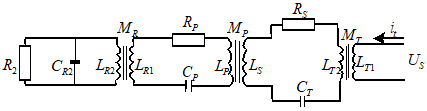Figure 7. The process of electrical signal conditioning

5. Experimental Verification

To prove the feasibility of our method, an energy-carrying communication experimental platform was constructed based on the ICPT system. The electrical signals were generated by STM32, and modulated by an adjustable voltage regulator circuit. The waveforms of the electrical signals and modulated signals were displayed by an oscilloscope with a coupling architecture, which contains an XL6009 voltage stabilization chip, an XKT412 inverter chip, and an LM393 voltage comparison chip. The control signal frequency and resonant frequency of the system were set to 1kHz and 38.165kHz, respectively.

The coils were mechanically wound with 17 turns. The electrical parameters of the coupling architecture are listed in Table 1.

Table 1. The electrical parameters of the coupling architecture

 Parameter Value Input DC voltage 5 Input DC current 1 Primary-side resonant capacitor 0.57 Primary-side resonant capacitor 47 Secondary-side resonant capacitor 0.51 Secondary-side resonant inductor 34

## 8.png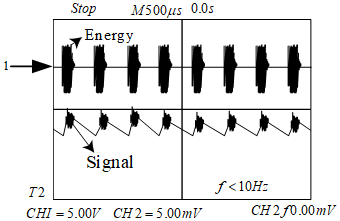Figure 8. The 1kHz control signals

As shown in Figure 8, the simulated transmission speed was 0.7764, when the system operated at 38.462kHz. Under the same frequency, the output voltage was 4.8V, output current was 0.78A, and the transmission speed was 0.7488. The experimental efficiency is lower than the simulation efficiency, because the electrical parameters of the variation in the coupling architecture in the high-frequency circuit brings excessive losses. To improve the transmission efficiency, the resonant frequency should be increased by redesigning the coils.

Experimental analysis shows that our method can effectively modulate the electrical signals into energy carrier signals, and complete the RST of electrical signals, without increasing the difficulty of system design.

5.1 Signal transmission delay

To compare the control effects of different methods, the RST delays of electrical signals of our method at different intensities of electromagnetic interference were compared with those of the fully coupled capacitor (FCC) model and empirical wavelet transform (EWT).

Table 2. The RST delays of different methods

 Interference intensity /μT RST delay/ms FCC EWT Our method 0 25 32 0 0.4 34 46 0 0.8 46 66 0 1.2 52 89 1 1.6 88 120 2 2.0 168 132 5

As shown in Table 2, the RST delays of all three methods decreased with the growing electromagnetic interference. When the interference intensity was 0 (i.e. no interference), the RST delay of FCC was 25ms, that of EWT was 32ms, and that of our method was 0. When the interference intensity increased to 2.0μT, the RST delay of FCC was 168ms, that of EWT was 132ms, and that of our method was merely 5ms. Therefore, the transmission delay of our method is so small as to be negligible under strong interference. This fully demonstrates the RST control ability of our method for electrical signals.

6. Conclusions

To mitigate the impacts of the transmission on the frequency of electrical signals, this paper proposes an RST method for electrical signals based on parallel injection and series pickup. By this method, the RST of electrical signals is realized through parallel injection and series pickup. The following conclusions can be drawn from the experimental results:

(1) When the system operated at 38.462kHz, the simulated transmission speed was 0.7764, while the experimental transmission speed was 0.7488. This means our method can effectively modulate the electrical signals into energy carrier signals, and complete the RST of electrical signals.

(2) When the interference intensity increased to 2.0μT, the RST delay of our method was merely 5ms. This fully demonstrates that our method can effectively reduce the transmission delay under strong interference, and implement the RST control of electrical signals.

Of course, there are some limitations of our research. For example, the electrical signals are easily lost during the transmission, owing to their instability. The future research will introduce real-time detection and intelligent control to the proposed method, aiming to detect and regulate the signal states in real time, and to avoid signal loss.

Acknowledgment

This work was supported by Science and Technology Breakthrough Project of Henan Provincial Science and Technology Department under the program “Brain Tumor Image Segmentation Based on Depth Learning” (Grant No.: 182102310694) and “Energy Consumption Modeling and Measurement in Virtual Environment” (Grant No.: 182102310034), and Development Plan Project of Henan Provincial Science and Technology Department under the program “Research and Development of University Financial Intelligent Platform in the Context of Cloud Computing” (Grant No.: 192400410368).

References

 Aysal, T.C., Barner, K.E. (2008). Blind decentralized estimation for bandwidth constrained wireless sensor networks. IEEE Transactions on Wireless Communications, 7(5): 1466-1471. https://doi.org/10.1109/TWC.2008.060687

 Hallez, H., De Vos, M., Vanrumste, B., Van Hese, P., Assecondi, S., Van Laere, K., Dupont, P., Van Paesschen, W., Van Huffel, S., Lemahieu, I. (2009). Removing muscle and eye artifacts using blind source separation techniques in ictal EEG source imaging. Clinical Neurophysiology, 120(7): 1262-1272. https://doi.org/10.1016/j.clinph.2009.05.010

 Nakajima, H., Nakadai, K., Hasegawa, Y., Tsujino, H. (2009). Blind source separation with parameter-free adaptive step-size method for robot audition. IEEE Transactions on Audio, Speech, and Language Processing, 18(6): 1476-1485. https://doi.org/10.1109/TASL.2009.2035219

 Rodriguez, A., Laio, A. (2014). Clustering by fast search and find of density peaks. Science, 344(6191): 1492-1496. https://doi.org/10.1126/science.1242072

 Hinton, G.E., Osindero, S., Teh, Y.W. (2006). A fast learning algorithm for deep belief nets. Neural Computation, 18(7): 1527-1554. https://doi.org/10.1162/neco.2006.18.7.1527

 Sangeetha, J., Jayasankar, T. (2019). Emotion speech recognition based on adaptive fractional deep belief network and reinforcement learning. In Cognitive Informatics and Soft Computing, pp. 165-174. https://doi.org/10.1007/978-981-13-0617-4_16

 Srivastava, N., Hinton, G., Krizhevsky, A., Sutskever, I., Salakhutdinov, R. (2014). Dropout: A simple way to prevent neural networks from overfitting. The Journal of Machine Learning Research, 15(1): 1929-1958. https://doi.org/10.5555/2627435.2670313

 Hinton, G.E. (2012). A practical guide to training restricted Boltzmann machines. In Neural networks: Tricks of the Trade, pp. 599-619. https://doi.org/10.1007/978-3-642-35289-8_32

 Corsini, G., Mossa, A., Verrazzani, L. (1996). Signal-to-noise ratio and autocorrelation function of the image intensity in coherent systems. Sub-Rayleigh and super-Rayleigh conditions. IEEE Transactions on Image Processing, 5(1): 132-141. https://doi.org/10.1109/83.481677

 Yatsenko, V., Kolesnik, Y., Titarenko, T. (1994). Identification of the non-Gaussian chaotic dynamics of the radioemission back scattering processes. IFAC Proceedings Volumes, 27(8): 277-281. https://doi.org/10.1016/S1474-6670(17)47728-7

 Leung, H., Lo, T. (1993). Chaotic radar signal processing over the sea. IEEE Journal of Oceanic Engineering, 18(3): 287-295. https://doi.org/10.1109/JOE.1993.236367

 Mukherjee, S., Osuna, E., Girosi, F. (1997). Nonlinear prediction of chaotic time series using support vector machines. In Neural Networks for Signal Processing VII. Proceedings of the 1997 IEEE Signal Processing Society Workshop, pp. 511-520. https://doi.org/10.1109/NNSP.1997.622433

 Leung, H., Hennessey, G., Drosopoulos, A. (2000). Signal detection using the radial basis function coupled map lattice. IEEE Transactions on Neural Networks, 11(5): 1133-1151. https://doi.org/10.1109/72.870045

 Sakai, K., Noguchi, Y., Asada, S.I. (2008). Detecting chaos in a citrus orchard: Reconstruction of nonlinear dynamics from very short ecological time series. Chaos, Solitons & Fractals, 38(5): 1274-1282. https://doi.org/10.1016/j.chaos.2007.01.144

 Zadeh, A.E. (2010). Automatic recognition of radio signals using a hybrid intelligent technique. Expert Systems with Applications, 37(8): 5803-5812. https://doi.org/10.1016/j.eswa.2010.02.027

 Kugiumtzis, D. (1996). State space reconstruction parameters in the analysis of chaotic time series—the role of the time window length. Physica D: Nonlinear Phenomena, 95(1): 13-28. https://doi.org/10.1016/0167-2789(96)00054-1

 Pépin, L., Kuntz, P., Blanchard, J., Guillet, F., Suignard, P. (2017). Visual analytics for exploring topic long-term evolution and detecting weak signals in company targeted tweets. Computers & Industrial Engineering, 112: 450-458. https://doi.org/10.1016/j.cie.2017.01.025

 Chang, C.C., Lin, C.J. (2011). LIBSVM: A library for support vector machines. ACM Transactions on Intelligent Systems and Technology (TIST), 2(3): 1-27. https://doi.org/10.1145/1961189.1961199